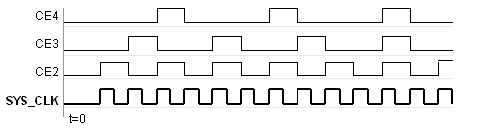# Timing and Clocking - 2022.1 English

Document ID
UG1483
Release Date
2022-05-26
Version
2022.1 English

## Discrete Time Systems

Designs in Model Composer are discrete time systems. In other words, the signals and the blocks that produce them have associated sample rates. A block’s sample rate determines how often the block is awoken (allowing its state to be updated). Model Composer sets most sample rates automatically. A few blocks, however, set sample rates explicitly or implicitly.

Note: For an in-depth explanation of Simulink discrete time systems and sample times, consult the Using Simulink reference manual from the MathWorks, Inc.

A simple Model Composer model illustrates the behavior of discrete time systems. Consider the model shown below. It contains a gateway that is driven by a Simulink source (Sine Wave), and a second gateway that drives a Simulink sink (Scope).

Figure 1. Discrete Time System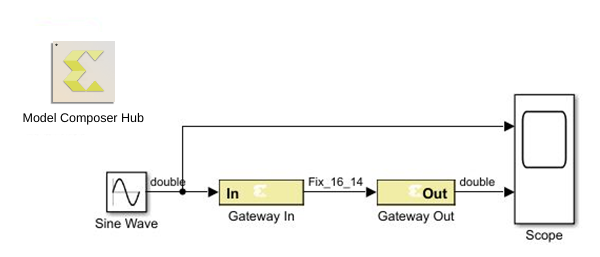The Gateway In block is configured with a sample period of one second. The Gateway Out block converts the Xilinx fixed-point signal back to a double (so it can analyzed in the Simulink scope), but does not alter sample rates. The scope output below shows the unaltered and sampled versions of the sine wave.

Figure 2. Scope Output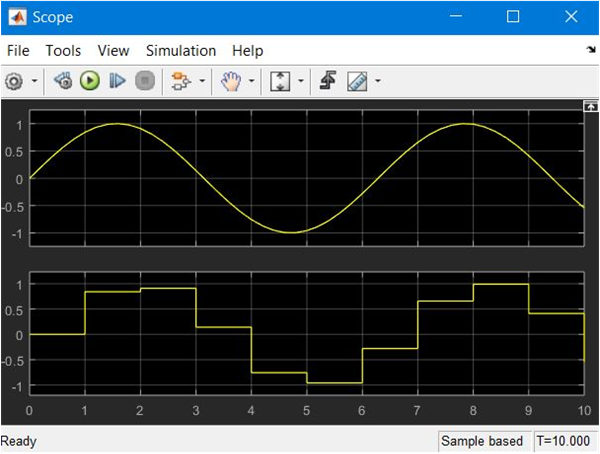## Multirate Models

Model Composer supports multirate designs, i.e., designs having signals running at several sample rates. Model Composer automatically compiles multirate models into hardware. This allows multirate designs to be implemented in a way that is both natural and straightforward in Simulink.

## Rate-Changing Blocks

The Model Composer HDL library includes blocks that change sample rates. The most basic rate changers are the Up Sample and Down Sample blocks. As shown in the following figure, these blocks explicitly change the rate of a signal by a fixed multiple that is specified in the block’s dialog box.

Figure 3. Rate Change Dialog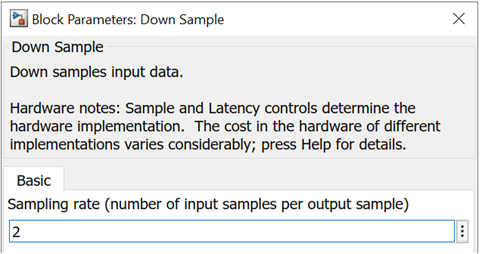Other blocks (e.g., the Parallel To Serial and Serial To Parallel converters) change rates implicitly in a way determined by block parameterization.

Consider the simple multirate example below. This model has two sample periods, SP1 and SP2. The Gateway In dialog box defines the sample period SP1. The Down Sample block causes a rate change in the model, creating a new rate SP2 which is half as fast as SP1.

Figure 4. Multirate Example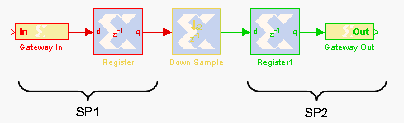## Hardware Oversampling

Some HDL blocks are oversampled, i.e., their internal processing is done at a rate that is faster than their data rates. In hardware, this means that the block requires more than one clock cycle to process a data sample. In Simulink such blocks do not have an observable effect on sample rates.

Although blocks that are oversampled do not cause an explicit sample rate change in Simulink, Model Composer considers the internal block rate along with all other sample rates when generating clocking logic for the hardware implementation. This means that you must consider the internal processing rates of oversampled blocks when you specify the Simulink system period value in the System Generator token dialog box.

## Asynchronous Clocking

Model Composer focuses on the design of hardware that is synchronous to a single clock. It can, under some circumstances, be used to design systems that contain more than one clock. This is possible provided the design can be partitioned into individual clock domains with the exchange of information between domains being regulated by dual port memories and FIFOs. The remainder of this topic focuses exclusively on the clock-synchronous aspects of Model Composer. This discussion is relevant to both single-clock and multiple-clock designs.

## Synchronous Clocking

By default, Model Composer creates designs with synchronous clocking, where multiple rates are realized using clock enables. When Model Composer compiles a model into hardware, it preserves the sample rate information of the design in such a way that corresponding portions in hardware run at appropriate rates. In hardware, Model Composer generates related rates by using a single clock in conjunction with clock enables, one enable per rate. The period of each clock enable is an integer multiple of the period of the system clock.

Inside Simulink, neither clocks nor clock enables are required as explicit signals in a Model Composer design. When Model Composer compiles a design into hardware, it uses the sample rates in the design to deduce what clock enables are needed. To do this, it employs two user-specified values from the System Generator token: the Simulink system period and FPGA clock period. These numbers define the scaling factor between time in a Simulink simulation, and time in the actual hardware implementation. The Simulink system period must be the greatest common divisor (gcd) of the sample periods that appear in the model, and the FPGA clock period is the period, in nanoseconds, of the system clock. If p represents the Simulink system period, and c represents the FPGA system clock period, then something that takes kp units of time in Simulink takes k ticks of the system clock (hence kc nanoseconds) in hardware.

To illustrate this point, consider a model that has three Simulink sample periods 2, 3, and 4. The gcd of these sample periods is 1, and should be specified as such in the Simulink system period field for the model. Assume the FPGA clock period is specified to be 10 ns. With this information, the corresponding clock enable periods can be determined in hardware.

In hardware, refer to the clock enables corresponding to the Simulink sample periods 2, 3, and 4 as CE2, CE3, and CE4, respectively. The relationship of each clock enable period to the system clock period can be determined by dividing the corresponding Simulink sample period by the Simulink System Period value. Thus, the periods for CE2, CE3, and CE4 equal 2, 3, and 4 system clock periods, respectively. A timing diagram for the example clock enable signals is shown in the following figure.

Figure 5. Timing Diagram